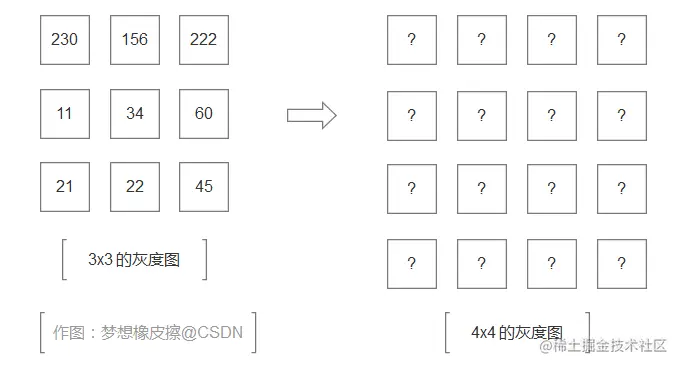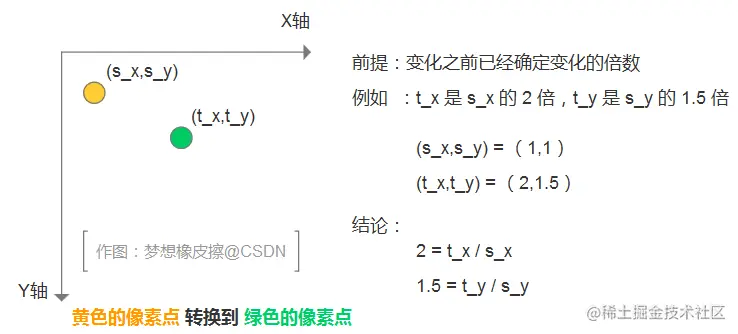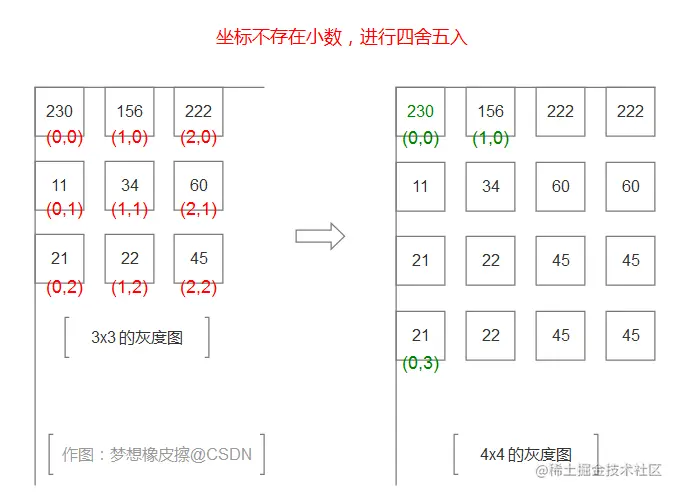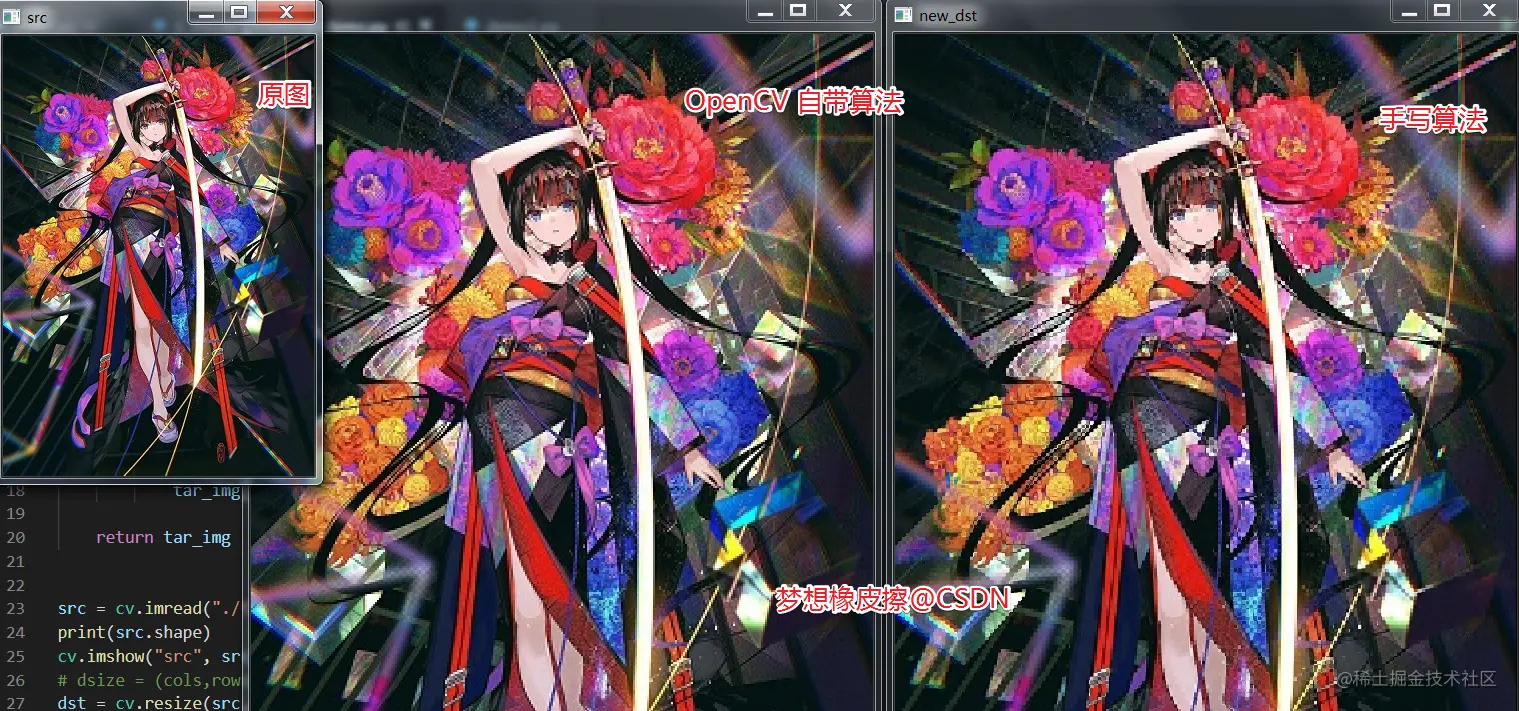# For the geometric transformation of Python OpenCV image, let's first talk about the extraordinary resize function

2022-01-30 03:05:14

Little knowledge , Great challenge ！ This article is participating in 「 A programmer must have a little knowledge 」 Creative activities

This article has participated in  「 Digging force Star Program 」 , Win a creative gift bag , Challenge creation incentive fund .

Python OpenCV 365 Day study plan , Go into the field of image with the eraser . This blog is the third in this series 41 piece .

## Basic knowledge

stay OpenCV Scaling is a common geometric transformation in , affine , Perspective transformation , I've learned about scaling functions in the previous section , Today, I'm going to review the old knowledge , While learning new knowledge .

Let's look at the function prototype corresponding to the three geometric transformations ：

``````dst = cv2.resize(src, dsize[, dst[, fx[, fy[, interpolation]]]])
dst = cv2.warpAffine(src, M, dsize[, dst[, flags[, borderMode[, borderValue]]]])
dst = cv2.warpPerspective(src, M, dsize[, dst[, flags[, borderMode[, borderValue]]]])
Copy code ``````

Let's start with zoom , The function is called `cv2.resize()`, Non null parameters have 2 individual , Namely `src` And `dsize`, It means the size of the source image and the scaled image .

``````import cv2 as cv

cv.imshow("src", src)
dst = cv.resize(src, (200, 200))
cv.imshow("dst", dst)
cv.waitKey(0)
cv.destroyAllWindows()
Copy code ``````

The above is the simplest code , The operation effect is as follows , A simple change has been madeThere is a common mistake in this case , Scaled values provide floating-point types , The error message is

``````TypeError: integer argument expected, got float
Copy code ``````

What's more, we need to pay attention to , Tuples `dsize` The two values of are explained as follows , Don't mistake the order .

``````# dsize = (cols,rows)  chinese ,( Width , Height )
dst = cv.resize(src, (400, 200))
Copy code ``````

This is a place to delve into , In fact, there are many details that need to be remembered , For example, in Cartesian coordinates , Record a coordinate point first x Axis , after y Axis , But in the computer , The image is saved in the form of a matrix , First, then , therefore ` wide x high x passageway ` Your pictures will be saved in ` high x wide x passageway ` In a three digit array of , In image processing , This is the ` high x wide x passageway ` memory , For example, through `shape` Get shape .

``````src = cv.imread("./t1.jpg")
print(src.shape)
Copy code ``````

The output is zero `( high , wide , passageway )`, But at this point `resize` Function does not comply with , It still uses `( wide , high )` Set up .

`fx`,`fy` For image `x`,`y` Scale in direction , Use this parameter , It is necessary to advance `dsize` Set to `(0,0)`, The test code is as follows ：

``````import cv2 as cv

print(src.shape)
cv.imshow("src", src)
# dsize = (cols,rows)  chinese ,( Width , Height )
dst = cv.resize(src, (0, 0),fx=0.5,fy=0.5)
cv.imshow("dst", dst)
cv.waitKey(0)
cv.destroyAllWindows()
Copy code ``````

The test result is , If not set up in advance `dsize` by `(0,0)`, that `fx` And `fy` Will not enter into force . Be careful `dsize=(0,0)`, If the data type is not right , The following error occurs ：

``````SystemError: new style getargs format but argument is not a tuple
Copy code ``````## interplolation The interpolation method when scaling

interplolation For the interpolation when scaling , There are several ways , These are the key points to explore today .

• `cv.INTER_NEAREST`： Nearest neighbor interpolation ;
• `cv.INTER_LINEAR`： Double line interpolation （ default setting ）;
• `cv.INTER_CUBIC`：4x4 Bicubic interpolation of pixel neighborhood ;
• `cv2.INTER_AREA`： Resampling based on local pixels . It may be the preferred method for image extraction , Because it produces cloudless texture results . But when the image zooms , It is similar to `INTER_NEAREST` Method .

### Nearest neighbor interpolation

This part is more energetic , So this one hour today , We're trying to get an interpolation algorithm , The nearest neighbor interpolation is good .

The idea of this algorithm is , Get the target pixel value through the pixel value . I found the most popular explanation in my study , Next, let me explain to you .

Let's say I have a 3x3 The gray image , We need to use the nearest neighbor interpolation algorithm , Get one 4x4 The gray image .First, through the coordinate system to understand the pixel in the zoom when the change .The final conclusion in the picture above is ：

• Of the target pixel x value = Original pixel x value * Multiple ;
• Of the target pixel y value = Original pixel y value * Multiple ;

What is the multiple of this case ？ It's easy to calculate , The original image is 3x3, Now it is 4x4, Where is the multiple `x,y` It's all about `4/3 = 0.75`.

Let's take a look at the operation and the result is as follows ：Here are the pixel values of two points , Take the target image `4x4 grayscale ` Medium `(3,0)` And `(3,3)` Two points to illustrate .

• `(3x0)` The value of the point is equal to `(3 x 0.75 ≈ 2,0 x 0.75 = 0)`, Original image `(2,0)` The color of the dot is `222`.
• `(3x3)` The value of the point is equal to `(3 x 0.75 ≈ 2,3 x 0.75 ≈ 2)`, Original image `(2,2)` The color of the dot is `45`;

After mastering the principle , You can implement this algorithm by yourself , Let's look at it first OpenCV Built in functions implement results .

``````import cv2 as cv
import numpy as np

#  Nearest neighbor interpolation algorithm , Source dream eraser  https://dream.blog.csdn.net/
def nearest_demo(src, multiple_x, multiple_y):
src_y, src_x, src_c = src.shape
tar_x, tar_y, tar_c = src_x*multiple_x, src_y*multiple_y, src_c
#  Generate a black target image
tar_img = np.zeros((tar_y, tar_x, tar_c), dtype=np.uint8)
print(tar_img.shape)
#  The value of the rendered pixel
#  Be careful  y  It's height ,x  It's the width
for y in range(tar_y-1):
for x in range(tar_x-1):
#  Calculate the new coordinates  (x,y)  What's the value of coordinates in the old graph
old_y = round(y/multiple_y)
old_x = round(x/multiple_x)
tar_img[y, x] = src[old_y, old_x]

return tar_img

print(src.shape)
cv.imshow("src", src)
# dsize = (cols,rows)  chinese ,( Width , Height )
dst = cv.resize(src, (0, 0), fx=2, fy=2, interpolation=cv.INTER_NEAREST)
cv.imshow("dst", dst)

new_dst = nearest_demo(src, 2, 2)
cv.imshow("new_dst", new_dst)

cv.waitKey(0)
cv.destroyAllWindows()
Copy code ``````

After running, it is found that the official algorithm is better .## Eraser bars

I hope today's 1 You get something in an hour , I'll see you on our next blog ~